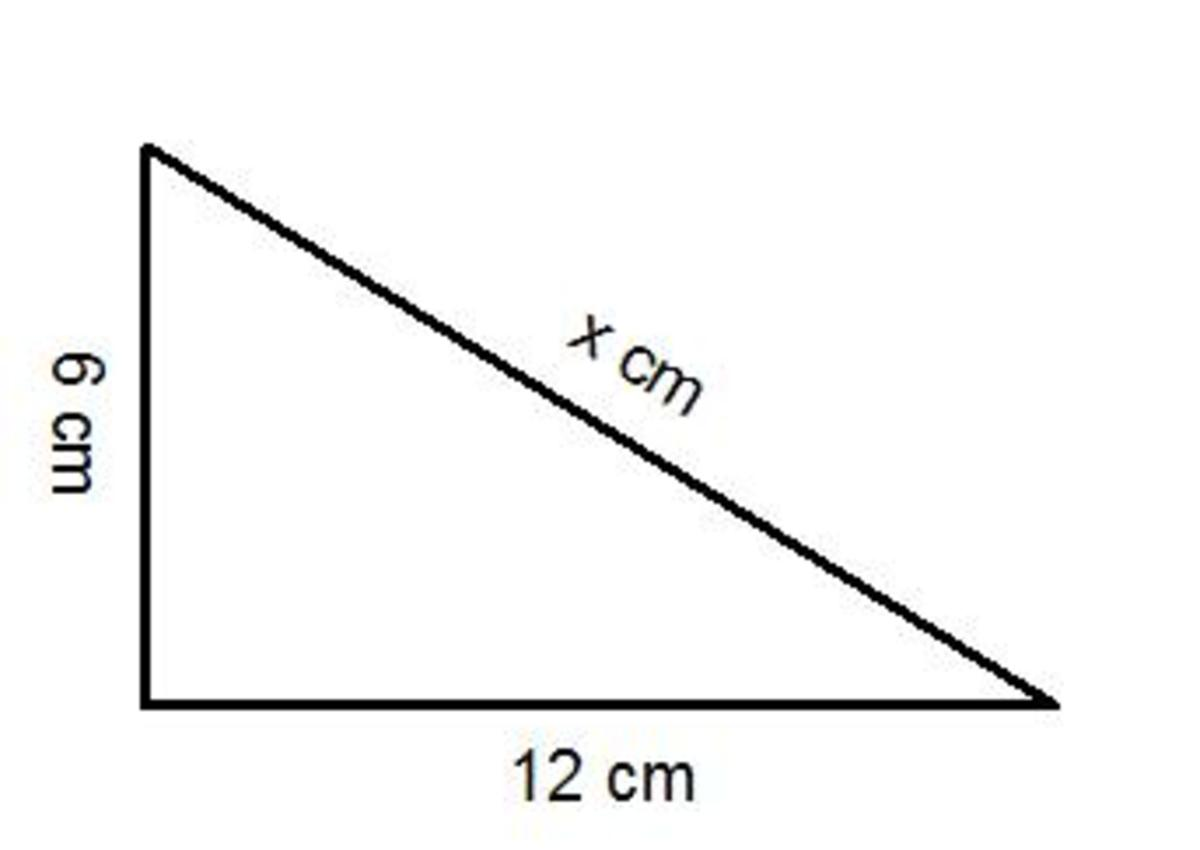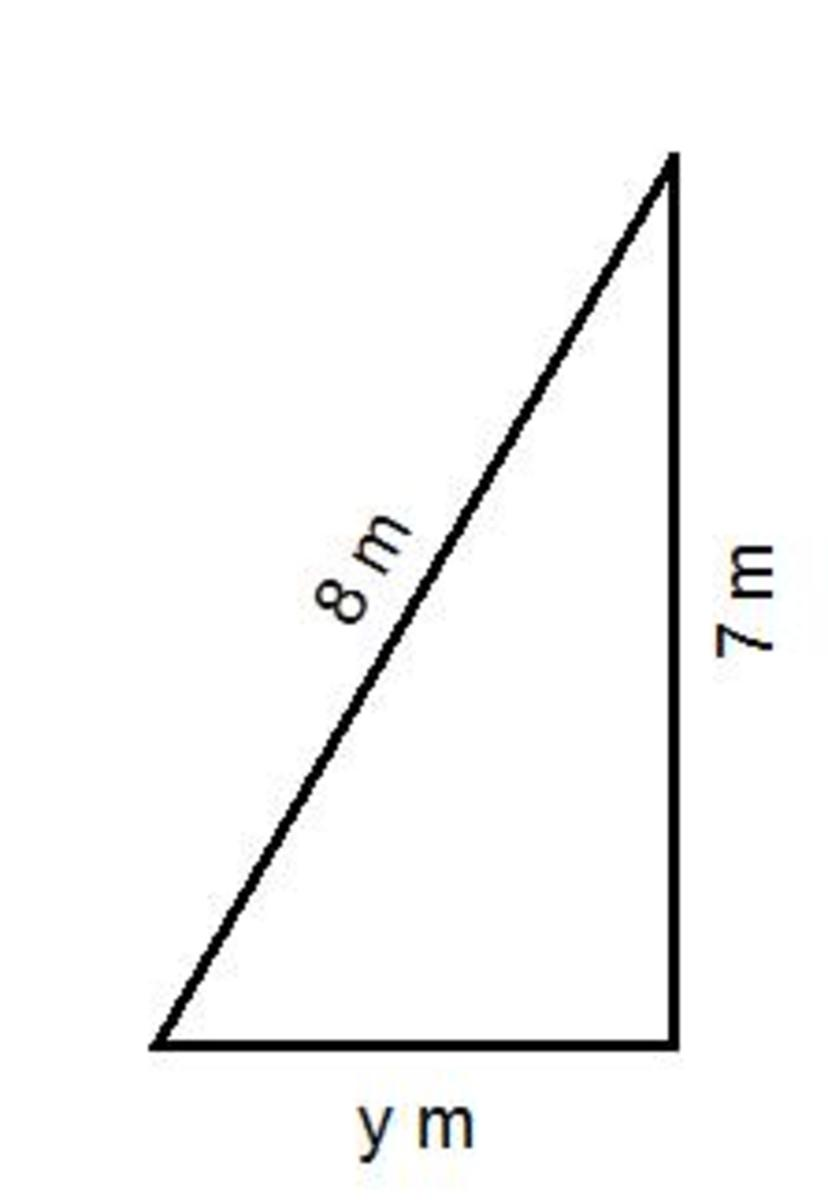Updated date:

# Pythagoras for dummies. A quick and easy guide to work out an unknown side using Pythagoras.

Author:

## Pythagoras Fast Method Video

What is Pythagoras used for?

Pythagoras (or Pythagoras Theorem) is used to calculate an unknown side length of a right angled triangle.

What information do you need to know to carry out Pythagoras?

You will need to know the lengths of 2 other sides. If you are given a side and an angle then the question is likely to be a trigonometry question.

Is Pythagoras complicated?

No, it can be done in 3 simple steps without using any algebra:

1) First square the two side lengths that are given in the question.

2) Either:

Add squares together if you are finding the longest side (aka the hypotenuse)

Or find the difference if you are not working out the longest side.

3) Square root the answer from step 2. You will probably have to use your calculator to do this.

What is the formula for Pythagoras?

a² + b² = c². Where a, b and c are the 3 sides with c being the longest side. If you are in a high math group then you should really use this formula. However, in this hub we will use the 3 steps above.

Example 1

Work out the length of the missing side in this right angled triangle (x).1) First square the two side lengths that are given in the question.

6² = 36

12² = 144

2) Either:

Add squares together if you are finding the longest side (aka the hypotenuse)

Or find the difference if you are not working out the longest side.

36 + 144 = 180 (Add them as x is the longest side)

3) Square root the answer from step 2. You will probably have to use your calculator to do this.

√180 = 13.4 cm to 1 decimal place.

Example 2

Calculate the length of the missing side in this right angled triangle (x).1) First square the two side lengths that are given in the question.

8² = 64

7² = 49

2) Either:

Add squares together if you are finding the longest side (aka the hypotenuse)

Or find the difference if you are not working out the longest side.

64 - 49 = 15 (Take them as y is not the longest side).

3) Square root the answer from step 2. You will probably have to use your calculator to do this.

√15 = 3.9 cm to 1 decimal place.

## Comments

ella on September 26, 2011:

thank god i have a test on this tomorrow and i had no idea what to do but now i do (:

MazioCreate on September 05, 2011:

Another simplified explanation to completing this formula. Thanks

Liam Hallam from Nottingham UK on September 05, 2011:

Nice hub catman, I still remember a² + b² = c² from school and it has so many real life uses too!